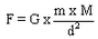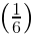Lakhmir Singh & Manjit Kaur: Gravitation, Solutions- 1

# Lakhmir Singh & Manjit Kaur: Gravitation, Solutions- 1 - Science Class 9

Page No - 100

Question 1:
What is the value of gravitational constant G (i) on the earth, and (ii) on the moon ?
Solution :
Value of gravitational constant G on the earth and the moon is = 6.67 x 10-11 Nm2/kg2
Note that the value of G always remains constant irrespective of the location.

Question 2:
Which force is responsible for the moon revolving round the earth ?
Solution :
Gravitational force is responsible for the moon revolving round the earth.

Question 3:
Does the acceleration produced in a freely falling body depend on the mass of the body ?
Solution :
No, the acceleration produced in a freely falling body is independent of the mass of the body.

Question 4:
Name the scientist who gave the three laws of planetary motion.
Solution :
Johannes Kepler gave the three laws of planetary motion.

Question 5:
Name the scientist who explained the motion of planets on the basis of gravitational force between the sun and planets.
Solution :
Newton explained the motion of planets on the basis of gravitational force between the sun and planets.

Question 6:
State the Kepler’s law which is represented by the relation r3 ∝ T2.
Solution :
Kepler’s law of periods states that: The cube of the mean distance of a planet from the sun is directly proportional to the square of time it takes to move around the sun.

Question 7:
Which of the Kepler’s laws of planetary motion led Newton to establish the inverse-square rule for gravitational force between two bodies ?
Solution :
Kepler’s third law of planetary motion led Newton to establish the inverse-square rule for gravitational force between two bodies.

Question 8:
Name the property of earth which is responsible for extremely small acceleration being produced in it as a result of attraction by other small objects.
Solution :
Extremely large mass of the earth.

Question 9:
What is the acceleration produced in a freely falling body of mass 10 kg ? (Neglect air resistance)
Solution :
Acceleration produced in a freely falling body, irrespective of its mass, is 9.8m/s2

Question 10:
When an object is dropped from a height, it accelerates and falls down. Name the force which accelerates the object.
Solution :
Gravitational force of the earth.

Question 11:
Give the formula for the gravitational force F between two bodies of masses M and m kept at a distance d from each other.
Solution :
The gravitational force F between two bodies of masses M and m kept at a distance d from each other is :Here, Gravitational Constant, G= 6.7x 10-11Nm2kg-2

Question 12:
What force is responsible for the earth revolving round the sun ?
Solution :
Gravitational force is responsible for the earth revolving round the sun.

Question 13:
What name has been given to the force with which two objects lying apart attract each other ?
Solution :
Gravitational force causes two objects lying apart attract each other.

Question 14:
What type of force is involved in the formation of tides in the sea ?
Solution :
Gravitational force (exerted mainly by the moon and to some extent by the sun) is involved in the formation of tides in the sea.

Question 15:
Which force is responsible for holding the solar system together ?
Solution :
Gravitational force of the sun holds the solar system together.

Question 16:
What is the weight of a 1 kilogram mass on the earth ? (g = 9.8 m/s2).
Solution :
Weight, W = m x g
= 1 kg x 9.8m/s2=9.8 N

Question 17:
On what factor/factors does the weight of a body depend ?
Solution :
The weight of a body is directly proportional to its mass. It also depends on the acceleration due to gravity which varies from place to place.

Question 18:
As the altitude of a body increases, do the weight and mass both vary ?
Solution :
Weight of the body varies with altitude; mass of an object is constant.

Question 19:
If the same body is taken to places having different gravitational field strength, then what will vary: its weight or mass ?
Solution :
Its weight varies; mass of an object is constant.

Question 20:
If the mass of an object be 10 kg, what is its weight ? (g = 9.8 m/s2).
Solution :
Weight, W = m x g = 10 x 9.8 =98 N

Question 21:
The weight of a body is 50 N. What is its mass ? (g = 9.8 m/s2).
Solution :
Weight, W = m x g

Question 22:
A body has a weight of 10 kg on the surface of earth. What will be its weight when taken to the centre of the earth ?
Solution :
Its weight will be zero as value of g is zero at the centre of the earth.

Question 23:
Write down the weight of a 50 kg mass on the earth, (g = 9.8 m/s2).
Solution :
Weight, W= m x g =50 x 9.8=490N

Question 24:
If the weight of a body on the earth is 6 N, what will it be on the moon ?
Solution :
Weight of the body on the surface of moon will be 1N approx. as the value of g on the surface of moon is one-sixth that of the earth

Question 25:
State whether the following statements are true or false :
(a) A falling stone also attracts the earth.
(b) The force of gravitation between two objects depends on the nature of medium between them.
(c) The value of G on the moon is about one-sixthof the value of G on the earth.(d) The acceleration due to gravity acting on a freely falling body is directly proportional to the mass of the body.(e) Tire weight of an object on the earth is about one-sixth of its weight on the moon.

Solution :
(a)True
(b)False
(c)False
(d)False
(e) False

The document Lakhmir Singh & Manjit Kaur: Gravitation, Solutions- 1 | Science Class 9 is a part of the Class 9 Course Science Class 9.
All you need of Class 9 at this link: Class 9

## Science Class 9

66 videos|355 docs|97 tests

## FAQs on Lakhmir Singh & Manjit Kaur: Gravitation, Solutions- 1 - Science Class 9

 1. What is gravitation?Ans. Gravitation is the force of attraction that exists between any two objects with mass. It is responsible for the phenomenon of weight and keeps celestial bodies like planets, moons, and stars in their orbits.
 2. What is the universal law of gravitation?Ans. The universal law of gravitation states that every particle in the universe attracts every other particle with a force that is directly proportional to the product of their masses and inversely proportional to the square of the distance between their centers.
 3. How does the force of gravity affect objects on Earth?Ans. The force of gravity pulls objects towards the center of the Earth, giving them weight. It is responsible for keeping objects grounded and causes them to fall when released from a height. The acceleration due to gravity on Earth is approximately 9.8 m/s².
 4. What are the factors that affect the gravitational force between two objects?Ans. The gravitational force between two objects depends on two factors: the mass of the objects and the distance between their centers. The greater the mass of the objects, the stronger the gravitational force. Similarly, the closer the objects are to each other, the stronger the gravitational force.
 5. How does the force of gravity explain the motion of planets in their orbits?Ans. The force of gravity between the Sun and planets is responsible for the motion of planets in their orbits. The gravitational force acts as a centripetal force, continuously pulling the planets towards the Sun. This force allows planets to maintain their elliptical orbits around the Sun.

## Science Class 9

66 videos|355 docs|97 testsExplore Courses for Class 9 examSignup to see your scores go up within 7 days! Learn & Practice with 1000+ FREE Notes, Videos & Tests.
10M+ students study on EduRev
Track your progress, build streaks, highlight & save important lessons and more!
Related Searches

,

,

,

,

,

,

,

,

,

,

,

,

,

,

,

,

,

,

,

,

,

,

,

,

;